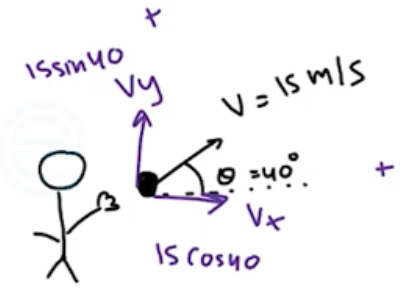Need Help?

Subscribe to Physics 1

###### \${selected_topic_name}
• Notes
• Comments & Questions

$\begin{array}{l}{\text { (a) What is the magnitude of the momentum of a } 10,000-\mathrm{kg}} \\ {\text { truck whose speed is } 12.0 \mathrm{m} / \mathrm{s} ? \text { (b) What speed would a } 2000-\mathrm{kg}} \\ {\text { SUV have to attain in order to have (i) the same momentum? }} \\ {\text { (ii) the same kinetic energy? }}\end{array}$

$m=1000 \mathrm{kg}, \quad \mathrm{V}=12 \mathrm{m} / \mathrm{s}$

$p=m \cdot v=10000 * 12=1.2 * 10^{5} kg \ m/s$

$m=2000 k g \quad p=1.2 * 10^{5} \mathrm{kg} \ \mathrm{m/s} \quad \mathrm{V} ??$

$p=m \cdot v \longrightarrow V_{s u v} \frac{p}{m}=\frac{1.2 * 10^{5}}{2000}=60 \mathrm{m} / \mathrm{s}$

$K_{T}=K_{s u v}$

$\frac{1}{2} m_{T} {v_{T}}^{2}=\frac{1}{2} m_{\text {Suv }} {V_{\text {suv }}}^{2} \rightarrow V_{suv}=\sqrt{\frac{m_{T} {v_{T}}^{2}}{m_{suv}}}$

$V_{\text {suv }}=\sqrt{\frac{10000 *(12)^ 2}{2000}}=26.8 \mathrm{m} / \mathrm{s}$

$\begin{array}{l}{\text { In a certain men's track and field event, the shotput has }} \\ {\text { a mass of } 7.30 \mathrm{kg} \text { and is released with a speed of } 15.0 \mathrm{m} / \mathrm{s} \text { at }} \\ {40.0^{\circ} \text { above the horizontal over a man's straight left leg. What are }} \\ {\text { the initial horizontal and vertical components of the momentum of }} \\ {\text { this shotput? }}\end{array}$$V_{x}=V \cos \theta=15 \cos 40=11.49 \mathrm{m/s}$

$V _y=V \sin \theta=15 \sin 40=9.642 \mathrm{m/s}$

$P_{x}=m v_{x}=7.3 * 11.49=83.9 \mathrm{kg} . \mathrm{m} / \mathrm{s}$

$P_{y}=m v_ y=7.3 * 9.642=70.4 \mathrm{kg} \cdot \mathrm{m} / \mathrm{s}$

$\text { A } 0.0450 \text { -kg golf ball initially at }$

$\begin{array}{l}{\text { rest is given a speed of } 25.0 \mathrm{m} / \mathrm{s} \text { when a club strikes. If the club }} \\ {\text { and ball are in contact for } 2.00 \mathrm{ms} \text { , what average force acts on the }} \\ {\text { ball? Is the effect of the ball's weight during the time of contact }} \\ {\text { significant? Why or why not? }}\end{array}$

$m=0.045 \quad k g$

$v_{0}=0$

$V_{2}=2 5 \mathrm{m} / \mathrm{s}$

$t=2 m s$

$f_{\text {avg }}??$

$J=F_{avg} \Delta t$

$J=P_{1}-P_{0}=m V_{1}-mV_{0}$

$F\text { avg } \Delta t=m v_{2} \rightarrow F_{\text {avg }}=\frac{m v_{2}}{\Delta t}=\frac{0.045 * 25}{2 * 10^{-3}}=562 \mathrm{N}$

$w=m g=0.045*9.8=0.441 N$

$\begin{array}{l}{\text { A } 0.160-\mathrm{kg} \text { hockey puck is moving on an icy, frictionless, }} \\ {\text { horizontal surface. At } t=0 \text { , the puck is moving to the right at }} \\ {3.00 \mathrm{m} / \mathrm{s} \text { . (a) Calculate the velocity of the puck (magnitude and }} \\ {\text { direction) after a force of } 25.0 \mathrm{N} \text { directed to the right has been }} \\ {\text { applied for } 0.050 \mathrm{s} \text { . (b) If, instead, a force of } 12.0 \mathrm{N} \text { directed to the }} \\ {\text { left is applied from } t=0 \text { to } t=0.050 \mathrm{s} \text { , what is the final velocity }} \\ {\text { of the puck? }}\end{array}$

$m=0.16 k g$

$F=25 N$

$\Delta t=0.05s$

$V_{1}=3 \mathrm{m} / \mathrm{s} \quad \longrightarrow$

$V_{2}= \quad ? ?$

$J_{x}=P_{2 x}-P_{1x} \quad ,P=m v$

$J_{x}=F_{x} \Delta t \rightarrow F_{x}\left(t_{2}-t_1\right)=25*0.05=1.25 \mathrm{kg} \cdot \mathrm{m} / \mathrm{s}$

$P_{2 x}=J_{x}+P_{1 x} \rightarrow P_{2 x}=1.25+(0.16)(3)=1.73 \mathrm{kg} . \mathrm{mls}$

$P_{2 x}=m v_{2} \rightarrow 1.73=0.16 \mathrm{v}_{2} \rightarrow \mathrm{v}_{2}=\frac{1.73}{0.16}=10.8 \mathrm{m} / \mathrm{s}$

$F=12 \mathrm{N} \leftarrow$

$J _x=F_{x}\left(t_{2}-t_{1}\right)=(-12)(0.05)=-0.6 \mathrm{kg} \cdot \mathrm{m} / \mathrm{s}$

$P_{2 x}=J_ x+P_{1x}=-0.6+(0.16)(3)=-0.12kg . \mathrm{m} / \mathrm{s}$

$v=P/{m}=\frac{-0.12}{0.16}=-0.75 \mathrm{m} / \mathrm{s} \leftarrow$

No comments yet